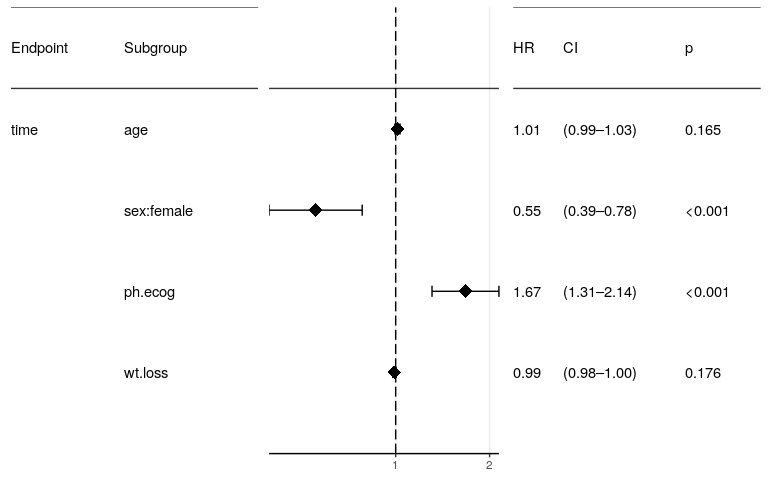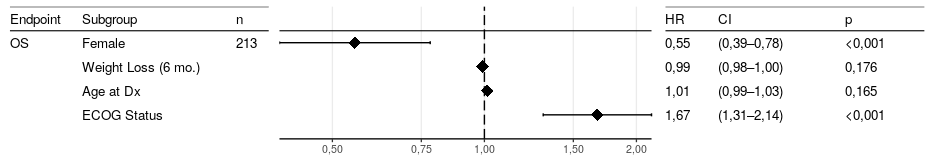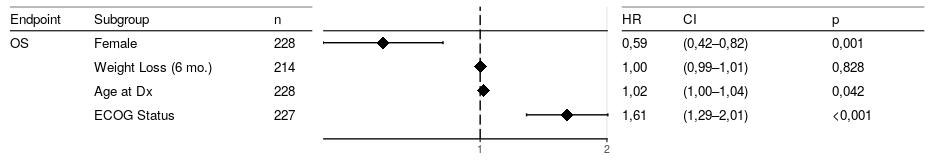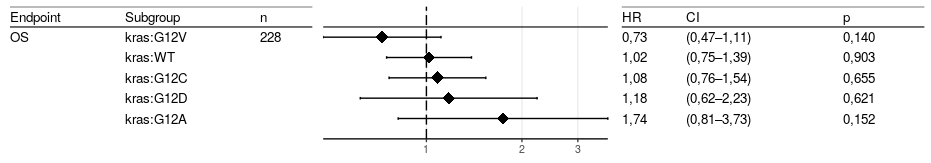# Multivariate Survival Analysis

#### 2019-02-12

For a very short introduction on survival data, please refer to the vignette on univariate analysis. Multivariate analysis, using the technique of Cox regression, is applied when there are multiple, potentially interacting covariates. While the log-rank test and Kaplan-Meier plots require categorical variables, Cox regression works with continuous variables. (Of course, you can use it with categorical variables as well, but this has implications which are described below.)

## The Multivariate Analysis result.

For the purpose of this vignette, we use the lung dataset from the survival package:

inst time status age sex ph.ecog ph.karno pat.karno meal.cal wt.loss
3 306 2 74 1 1 90 100 1175 NA
3 455 2 68 1 0 90 90 1225 15
3 1010 1 56 1 0 90 90 NA 15
5 210 2 57 1 1 90 60 1150 11
1 883 2 60 1 0 100 90 NA 0
12 1022 1 74 1 1 50 80 513 0
7 310 2 68 2 2 70 60 384 10
11 361 2 71 2 2 60 80 538 1
1 218 2 53 1 1 70 80 825 16
7 166 2 61 1 2 70 70 271 34

We load the tidyverse, for some tools the tidytidbits package and finally and the survivalAnalysis package:

library(tidyverse)
library(tidytidbits)
library(survivalAnalysis)

Possibly interesting covariates are patient age, sex, ECOG status and the amount of weight loss. Sex is encoded as a numerical vector, but is in fact categorical. We need to make it a factor. ECOG status is at least ordinally scaled, so we leave it numerical for now. Following the two-step philosophy of survivalAnalysis, we first perform the analysis with analyse_multivariate and store the result object. We provide readable labels for the covariates to allow easy interpretation. There is a print() implementation which prints essential information for our result.

covariate_names <- c(age="Age at Dx",
sex="Sex",
ph.ecog="ECOG Status",
wt.loss="Weight Loss (6 mo.)",
sex:female="Female",
ph.ecog:0="ECOG 0",
ph.ecog:1="ECOG 1",
ph.ecog:2="ECOG 2",
ph.ecog:3="ECOG 3")

survival::lung %>%
mutate(sex=rename_factor(sex, 1 = "male", 2 = "female")) %>%
analyse_multivariate(vars(time, status),
covariates = vars(age, sex, ph.ecog, wt.loss),
covariate_name_dict = covariate_names) ->
result
print(result)
#> Overall:
#>    n                 covariates Likelihood ratio test p Wald test p
#>  213 age, sex, ph.ecog, wt.loss                  <0.001      <0.001
#>  Score (logrank) test p
#>                  <0.001
#>
#> Hazard Ratios:
#>   factor.id         factor.name factor.value   HR Lower_CI Upper_CI Inv_HR
#>  sex:female                 Sex       female 0.55     0.39     0.78   1.81
#>     wt.loss Weight Loss (6 mo.) <continuous> 0.99     0.98      1.0   1.01
#>         age           Age at Dx <continuous> 1.01     0.99     1.03   0.99
#>     ph.ecog         ECOG Status <continuous> 1.67     1.31     2.14    0.6
#>  Inv_Lower_CI Inv_Upper_CI      p
#>          1.28         2.55 <0.001
#>           1.0         1.02  0.176
#>          0.97         1.01  0.165
#>          0.47         0.76 <0.001

## A Note on Categorical Variables

In the example above, you may have noted that the hazard ratio is given for women compared to men (women have a better outcome, HR 0.55). What is the hazard ratio for men compared to women? In the case of a binary variable, this is simply the inverted HR, which is also always provided (1.81). Things get more complicated with three or more categories. The rule is: You must choose one level of the factor as the reference level with defined hazard ratio 1.0. Then, for k levels, there will be k-1 pseudo variables created which represent the hazard ratio of this level compared to subjects in the reference level. (For the comparison of one level vs. all remaining subjects see the paragraph on one-hot analysis further down.)

As an example, we consider the two covariates which were significant above, sex and ECOG, and regard the ECOG status as a categorical variable with four levels. As reference level, we choose ECOG=0 with the parameter reference_level_dict.

survival::lung %>%
mutate(sex=rename_factor(sex, 1 = "male", 2 = "female"),
ph.ecog = as.factor(ph.ecog)) %>%
analyse_multivariate(vars(time, status),
covariates = vars(sex, ph.ecog),
covariate_name_dict=covariate_names,
reference_level_dict=c(ph.ecog="0"))
#> Overall:
#>    n   covariates Likelihood ratio test p Wald test p
#>  227 sex, ph.ecog                  <0.001      <0.001
#>  Score (logrank) test p
#>                  <0.001
#>
#> Hazard Ratios:
#>   factor.id factor.name factor.value   HR Lower_CI Upper_CI Inv_HR
#>  sex:female         Sex       female 0.58     0.42     0.81   1.72
#>   ph.ecog:1 ECOG Status            1 1.52     1.03     2.25   0.66
#>   ph.ecog:2 ECOG Status            2 2.58     1.66     4.01   0.39
#>   ph.ecog:3 ECOG Status            3 7.76     1.04    58.04   0.13
#>  Inv_Lower_CI Inv_Upper_CI      p
#>          1.24          2.4  0.001
#>          0.45         0.97  0.036
#>          0.25          0.6 <0.001
#>          0.02         0.96  0.046

Sidenote: We are very much used to see hazard ratios for a binary covariate. Please note the different interpretation for continous variables: The hazard ratio is to be interpreted with regard to a change of size 1. With a binary variable, there is only one step from 0 to 1. With age, the range is different! Assume we detect a hazard ratio of 1.04 for age in some study. What is the calculated HR of a 75y old patient compared to a 45y old?

exp((75-45)*log(1.04))
#>  3.243398

## A Forest Plot

The usual method to display results of multivariate analyses is the forest plot. The survivalAnalysis package provides an implementation which generates ready-to-publish plots and allows extensive customization.

forest_plot(result)Ok, this one is not ready to publish. We need to tweak some parameters:

• Adjust font size
• Change the figure size. The plot objects returned by forest_plot contain an attribute from a heuristic containing a suggested plot size (inches). This size is used for the following plot, hardcoded in the vignette. In your code, you can use tidytidbitssave_pdf, which reads this attribute. Further adjustment is trial&error. Please note that this is plotting and not text processing - if you specify a too small size, text will disappear or overlap.
• Correctly label the endpoint.
• Correctly label the subgroups. For technical reasons, this does not automatically use the covariate_name_dict passed to the analysis. In fact, the id displayed here is the same as the name for continuous covariates, but additionally contains the factor level for categorical covariates (“sex:female”). The covariate_names dictionary set up above already contains the additionally entries that we need.
• order by hazard ratio
• display the “n” count in the left table
• some more space for the plot, less space for the tables
• more breaks on the X axis
forest_plot(result,
factor_labeller = covariate_names,
endpoint_labeller = c(time="OS"),
orderer = ~order(HR),
labels_displayed = c("endpoint", "factor", "n"),
ggtheme = ggplot2::theme_bw(base_size = 10),
relative_widths = c(1, 1.5, 1),
HR_x_breaks = c(0.25, 0.5, 0.75, 1, 1.5, 2))The forest_plot function actually accepts any number of results and will display them all in the same plot. For example, you may want to display both OS and PFS in the same plot, but of course ordered and with a line separating the two. To do that,

• throw both results into forest_plot
• order first by endpoint, then, as you like, by something else: orderer = ~order(endpoint, factor.name)
• use a categorizer function returning a logical vector which determines where the separating line shall be drawn. For a flexible solution, the usual idiom is something like categorizer = ~!sequential_duplicates(endpoint, ordering = order(endpoint, factor.name)), where the ordering clause is identical to your orderer code.

Finally, if you want separate plots but display them in a grid, use forest_plot_grid to do the grid layout and forest_plot’s title argument to add the A, B, C… labels.

## Multiple Univariate Analyses

It is common practice to perform univariate analyses of all covariates first and take only those into the multivariate analysis which were significant to some level in the univariate analysis. (I see some pros and strong cons with this procedure, but am open to learn more on this). The univariate part can easily be achieved using purrr’s map function. A forest plot, as already said, will happily plot multiple results, even if they come as a list.


df <- survival::lung %>% mutate(sex=rename_factor(sex, 1 = "male", 2 = "female"))

map(vars(age, sex, ph.ecog, wt.loss), function(by)
{
analyse_multivariate(df,
vars(time, status),
covariates = list(by), # covariates expects a list
covariate_name_dict = covariate_names)
}) %>%
forest_plot(factor_labeller = covariate_names,
endpoint_labeller = c(time="OS"),
orderer = ~order(HR),
labels_displayed = c("endpoint", "factor", "n"),
ggtheme = ggplot2::theme_bw(base_size = 10))Note how the values only slighlty differ. The number of cases is larger each time because the multivariate analysis will only include the subset of subjects for which all covariates are known. Age is significant in UV but not in MV - there is probably interaction between ECOG and age.

## One-Hot Encoding

We are moving to the grounds of exploratory analysis. For a somewhat interesting example, we add the KRAS mutational status to the data set (by random sampling, for the sake of this tutorial). No, there is a categorical variable with five levels, but none of these comes natural as reference level. One may argue that wild type should be the reference level, but we may want to know if wild type is better than any KRAS mutation. If we omit wild-type and compare only among mutated tumors, there is definitely no suitable reference level.

The one-hot parameter triggers a mode where for each factor level, the hazard ratio vs. the remaining cohort is plotted. This means that no level is omitted. Please be aware of the statistical caveats. And please note that this has nothing to do any more with multivariate analysis. In fact, now you need the result of the univariate, categorically-minded analyse_survival.


survival::lung %>%
mutate(kras=sample(c("WT", "G12C", "G12V", "G12D", "G12A"),
nrow(.),
replace = T,
prob = c(0.6, 0.24, 0.16, 0.06, 0.04))
) %>%
analyse_survival(vars(time, status), by=kras) %>%
forest_plot(use_one_hot=TRUE,
endpoint_labeller = c(time="OS"),
orderer = ~order(HR),
labels_displayed = c("endpoint", "factor", "n"),
ggtheme = ggplot2::theme_bw(base_size = 10))We randomly assigned the RAS status, so results will differ at each generation of this vignette. If one of the subgroups should differ significantly, by the law of small numbers, it will probably be one of the rarer variants.